## HRC-I XRCF QE/Gain ratio maps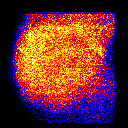B K183 eV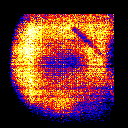O K525 eV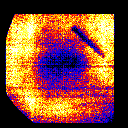Al K1487 eV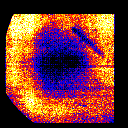Ti K4511 eV

## HRC-I Flight QE/Gain ratio maps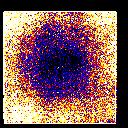B K183 eV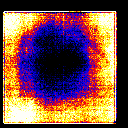C K277 eV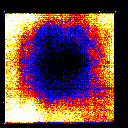O K525 eV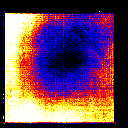Ni L852 eV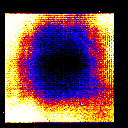Al K1487 eV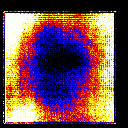Ag L2984 eV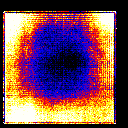Ti K4511 eV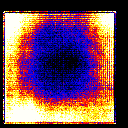Fe K6404 eV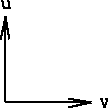## QE-Gain scatter plots

Scatter plots of the QE and Gain of each image/gain cell of SAC flat fields (blocking factor of 128), from within the CsI coated region (XRCF images were truncated to exclude the vignetted area). Due to the large number of data points (11772 for Flight, 8175 for XRCF), only 1/15 of the points are plotted, selected randomly. The QE values have been normalized, such that the mean of the central 12% of each flat field image is scaled to unity. Scaling factors were determined by eye, so that the curves for all energies are continuous.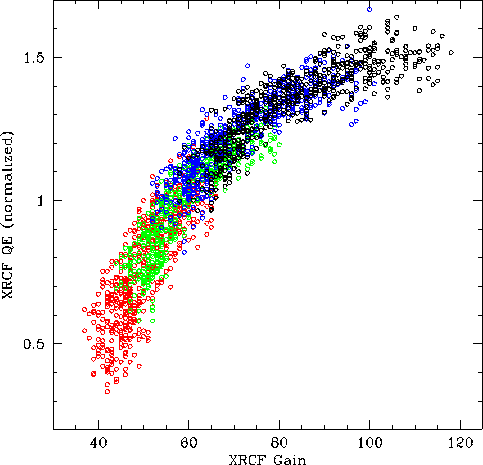B red scale factor = 1 O green scale factor = 1.2 Al blue scale factor = 1.4 Ti black scale factor = 1.5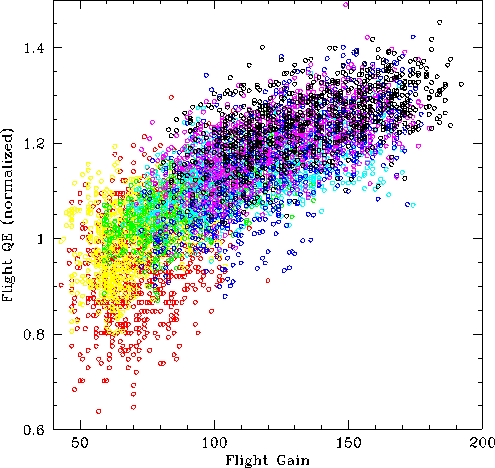B red scale factor = 1 C yellow scale factor = 1.05 O green scale factor = 1.15 Al cyan scale factor = 1.2 Ag blue scale factor = 1.25 Fe magenta scale factor = 1.27 Ti black scale factor = 1.3